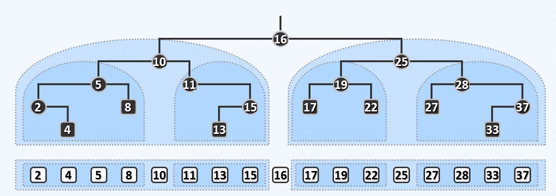HR
【单选题】What are the steps for deleting 16? 欲在以上二叉搜索树中删除节点16，可行的方案是：
A.
remove 16, make 25 the right child of 10 and 10 becomes the new root.将16摘除后令25为10的右子，从而10是新的根节点
B.
execute a zig operation on 16 making it no longer the root, and then remove it directly以16为轴进行一次zig操作使之不再是根节点，再直接摘除16
C.
exchange the key of node 16 and node 33, and remove the new node 16将节点16和节点33的关键码互换，再摘除新的节点16
D.
exchange the key of node 16 and node 15, remove the new node 16 and make 13 the right child of 11将节点16和节点15的关键码互换，摘除新的节点16并令13为11的右子手机使用分享复制链接新浪微博分享QQ微信扫一扫反馈A.

B.

C.

D.

A.

B.

C.

D.

A.
RF
B.
FF
C.
MF
D.
TG

A.
RF
B.
TG
C.
RJ
D.
MF

A.

B.

C.

D.

A.

B.

C.

D.

A.
0＜p≤1.6Mpa
B.
1.6MPa＜p≤10Mpa
C.
10MPa＜p≤100Mpa
D.
100MPa＜p≤1000MPa

A.
Y型过滤器
B.
U型过滤器
C.

D.

A.

B.

C.

D.

A.
0
B.
15
C.
20
D.
60
（ ）是指油品在规定的试验条件下，被冷却的试样能够流动的最低温度。
A.

B.

C.

D.

（ ）是指在规定条件下加热油品，逸出的油蒸气与空气形成混合气，当与火焰接触时能发生瞬间闪火时的最高温度。
A.

B.

C.

D.

A.
3和1
B.
4.5和2
C.
5和1
D.
4.5和1

A.

B.

C.

D.

《危险化学品安全管理条例》规定，生产.储存.使用剧毒化学品的单位，应当对本单位的生产.储存装置每年进行一次安全评价；生产.储存.使用其他危险化学品的单位，应当对本单位的生产.储存装置每（ ）年进行一次安全评价。
A.
B.
1
C.
2
D.
3

A.

B.

C.

D.

A.

B.

C.

D.

A.

B.

C.

D.

A.

B.

C.

D.

A.

B.

C.

D.

A.

B.

C.

D.

A.

B.

C.

D.

A.

B.

C.

D.

A.

B.

C.

D.

A.
1
B.
5
C.
10
D.
15

A.
1
B.
5
C.
10
D.
15

A.

B.

C.

D.

A.

B.

C.

D.

A.
20
B.
30
C.
50
D.
60

A.

B.

C.

D.

A.
1
B.
5
C.
2
D.
5

A.

B.

C.

D.

A.

B.

C.

D.

A.

B.

C.

D.

A.
100~200
B.
500~800
C.
1500~2000
D.
2000以上

A.
5
B.
3
C.
8
D.
2

A.

B.

C.

D.

A.
1
B.
3
C.
5
D.
7

A.
5
B.
3
C.
8
D.
2

A.

B.

C.

D.

A.
1
B.
4
C.
10
D.
20

A.
1/2
B.
1/3
C.
1/4
D.
1/5

A.

B.

C.

D.

A.
30
B.
20
C.
10
D.
40

A.
2
B.
5
C.
1
D.
3

A.
5
B.
10
C.
15
D.
20

A.

B.

C.

D.

A.

B.

C.

D.

A.
1万-5万
B.
6万-8万
C.
1万以下
D.
8万-12万
VL 型油轮载重吨（DWT）为（ ）。
A.
6万-8万
B.
12万-20万
C.
20万-30万
D.
30万以上

A.
1.852
B.
1.752
C.
1.952
D.
1.652

A.

B.

C.

D.

A.

B.

C.

D.

A.

B.

C.

D.

A.

B.

C.

D.

A.
2
B.
3
C.
4
D.
5

A.
DN400
B.
DN600
C.
DN300
D.
DN200

A.
13.8
B.
15.4
C.
17.1
D.
20.7

A.

B.

C.

D.

A.
9
B.
10
C.
11
D.
12

A.
50000m3
B.
10000~50000m3
C.
2500~10000m3
D.
500~2500m3

A.
D
B.
Z
C.
J
D.
Q

A.
H
B.
Z
C.
J
D.
G

A.

B.

C.

D.

A.

B.

C.

D.

A.

B.

C.

D.

A.

B.

C.

D.

（ ）的启闭件在外力作用下处于常闭状态，当设备或管道内的介质压力超过规定值时便自动开启，通过向系统外排放介质来降低管道或设备内压力。
A.

B.

C.

D.

A.
4
B.
5
C.
6
D.
7

A.
4
B.
5
C.
6
D.
7

A.
5
B.
7
C.
8
D.
10

A.
-30°~40°
B.
-45°~50°
C.
-50°~60°
D.
-55°~70°

A.
-30°~40°
B.
-45°~70°
C.
-50°~60°
D.
-55°~70°

A.
4
B.
5
C.
6
D.
7

A.

B.

C.

D.

A.
2
B.
3
C.
4
D.
5

A.
90%
B.
92%
C.
95%
D.
98%

A.
DN900
B.
DN600
C.
DN200
D.
DN1200

A.
RD
B.
C.
PN
D.
DN

A.
1
B.
2
C.
3
D.
4

A.

B.
1年
C.
2年
D.
4年

A.
1.2
B.
1.6
C.
10
D.
100

A.
442
B.
433
C.
422
D.
4222

A.

B.

C.

D.

A.
1
B.
2
C.
3
D.
4

A.

B.

C.

D.

A.
120
B.
60
C.
45
D.
30

A.

B.

C.

D.

A.
0.5
B.
1
C.
2
D.

A.
50mm
B.
80mm
C.
100mm
D.
120mm

A.
1~2
B.
2~3
C.
3~4
D.
4~5

A.

B.

C.

D.

A.

B.

C.

D.

A.
2
B.
5
C.
10
D.
15

A.
6
B.
7
C.
8
D.
9

A.
6
B.
7
C.
8
D.
9

A.

B.

C.

D.

A.
0.5
B.
1
C.
1.5
D.
2

A.

B.

C.

D.

A.

B.

C.

D.298%